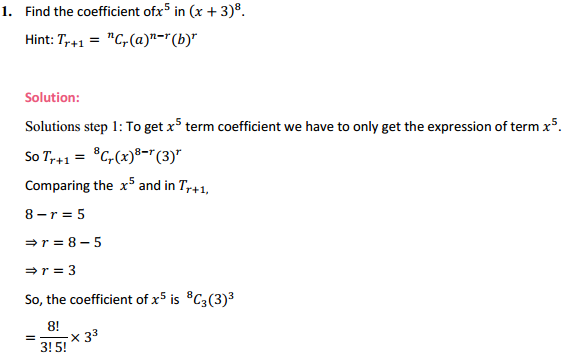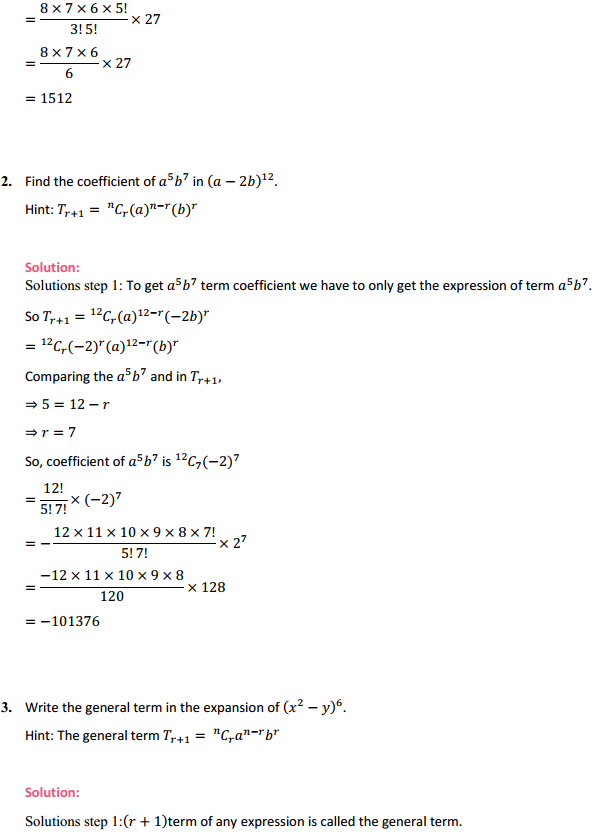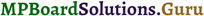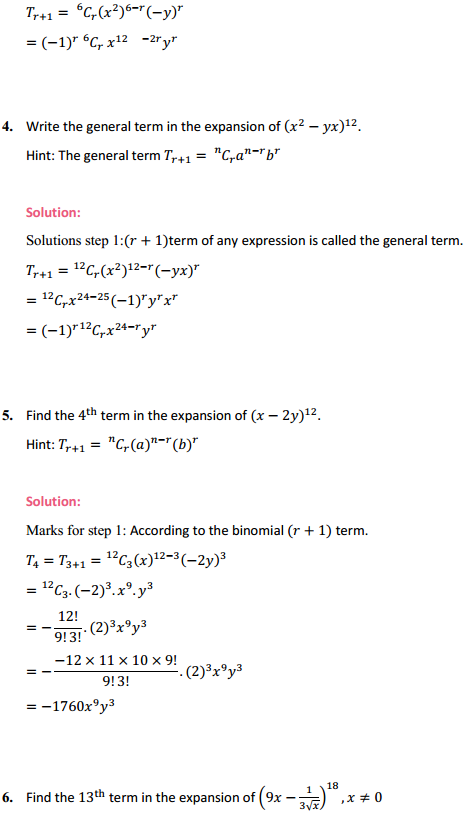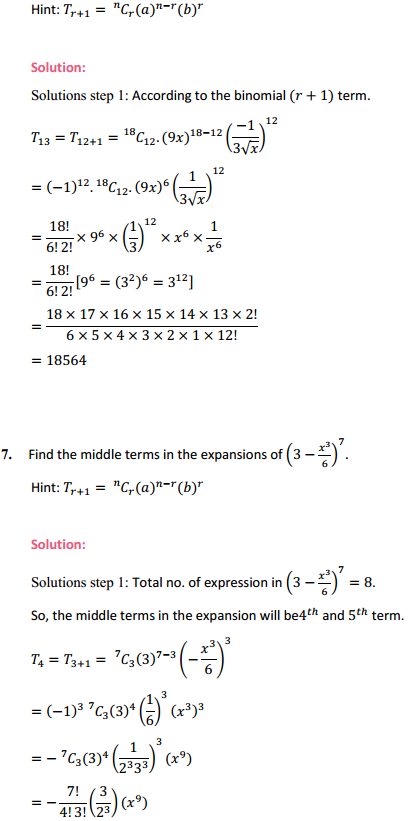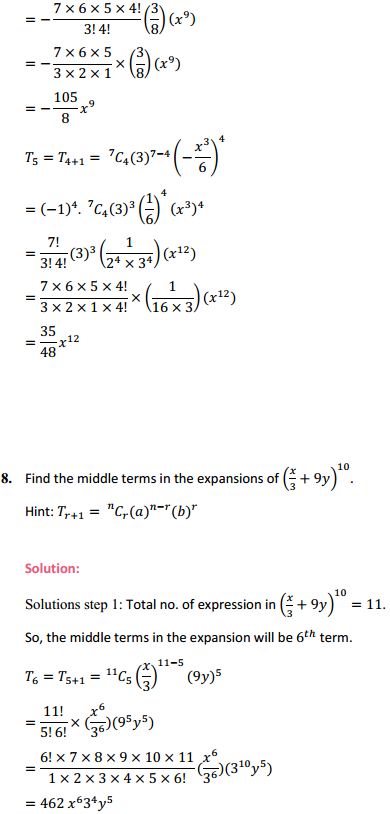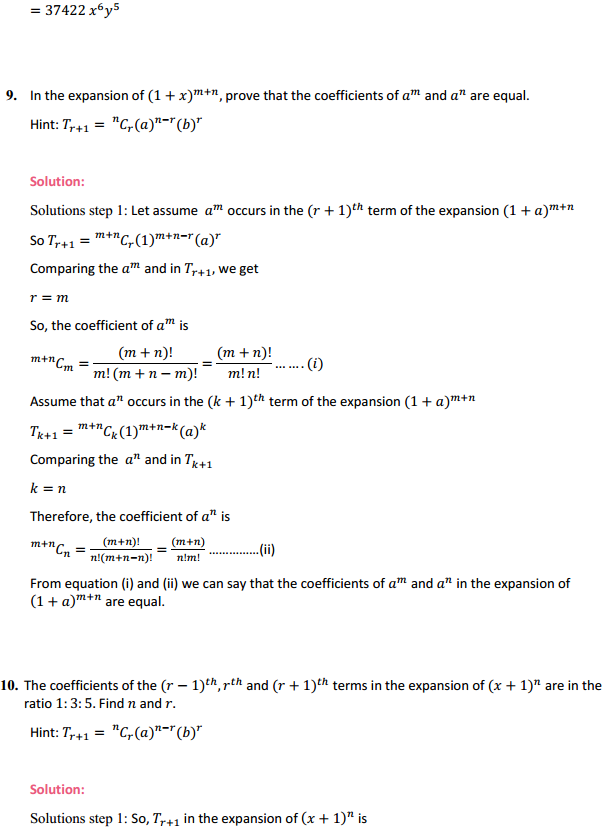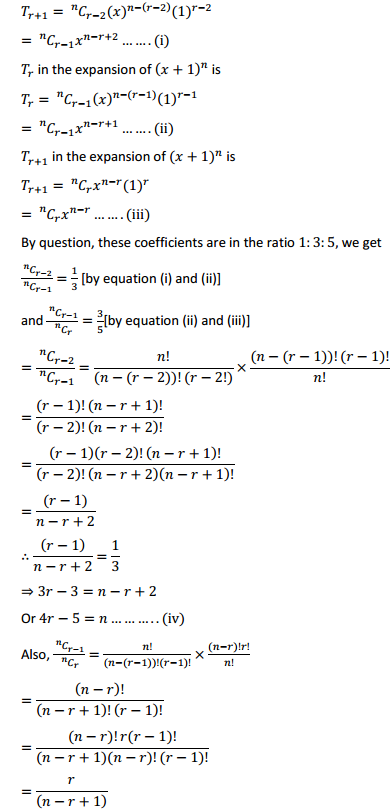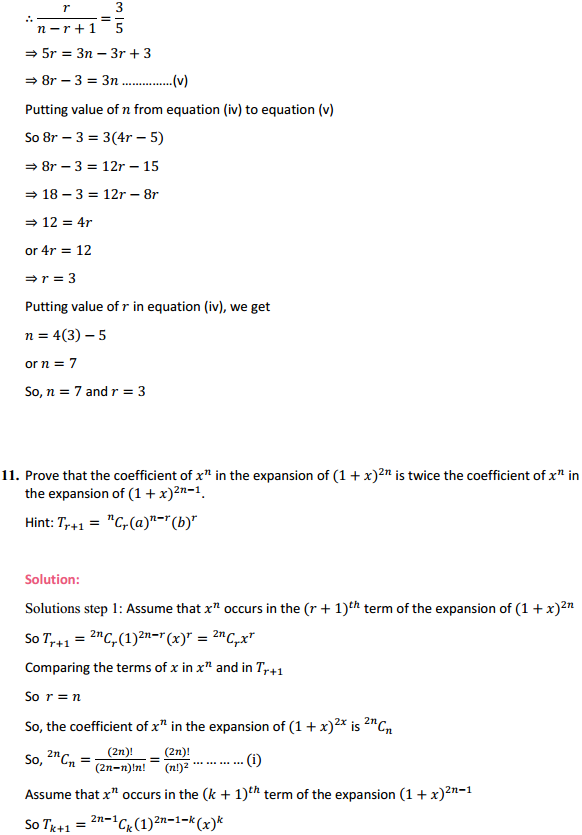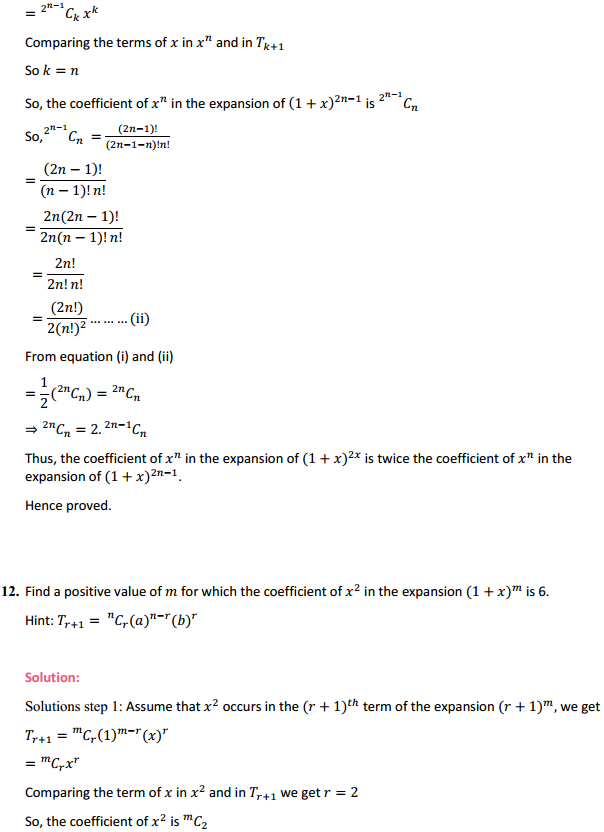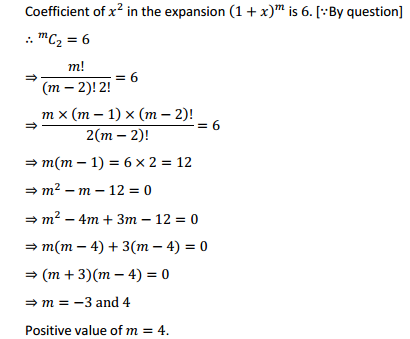# MP Board Class 11th Maths Solutions Chapter 8 Binomial Theorem Ex 8.2

In this article, we share MP Board Class 11th Maths Book Solutions Chapter 8 Binomial Theorem Ex 8.2.

## MP Board Class 11th Maths Solutions Chapter 8 Binomial Theorem Exercise 8.2